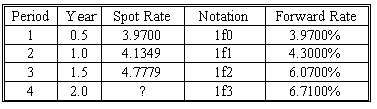### CFA Practice Question

There are 490 practice questions for this study session.

### CFA Practice Question

Kandy Miller is undecided about whether to purchase a four period bond or a series of one period bonds. Given the forward rates and spot rates provided below, what would Kandy earn on the four period spot rate bond?

Spot Rates and Six Month Forward Rates (Annualized Rates on a Bond Equivalent Basis)A. 5.2592
B. 3.6289
C. 6.0481
Explanation: While this looks like a "bootstrapping" problem, it is actually much easier. The spot rate is nothing more than all of the forward rates multiplied together and taken to the nth root. While the calculation is simpler than the bootstrapping problem, there are still a few areas for error. For example, each spot rate is annualized and needs to be divided by two to get the effective period rate. Equally important, after all of the forward rates have been multiplied by each other (being sure to add "1" to each rate before multiplying), remember to take the nth root (depending on the number of periods) and to subtract "1" afterwards. The detailed calculation is shown below:

({[(1+0.047779/2)3 x (1+0.0671/2)](1/4)}-1) x 2 =0.052592.

Note: In order to see the number in "percentage" form, it is necessary to multiply by 200 rather than by 2.

User Comment
kalps (1+r(3))(3) X (1+3f4)(1) = (1+r(4))(4) Same as spot rate calc but backwards
mtcfa Where did this formula come from???? This is at odds with the formula for computing spot rates on page 194 of the text.
chuong (1+Z4)^4 = (1+1f0)x(1+1f1)x(1+1f2)x(1+1f3)x(1+1f4)

Z4) = (1.0397)x(1.0430)x(1.0607)x(1.0671)^1/4 -1
gweiden This problem is much easier than it looks. You don't have to do any calculations. Simply follow the trend of the Forward Rates and Spot Rates. Since the spot rates are increasing moderatley compared to the forward rates, you know the the spot rate asked for must be higher than 4.7779. C doesn't make sense either since it is too much of an increase compared to the previous increases in spot rates. That leaves choice A.
Shelton answer from chuong is close except /2 piece
Janey i worked it out the same as gweiden
soukhov i solve it this way =)
6.71 / 6.07 * 4.77 = 5.273 -> A correct
nmech1984 @soukhov me too!